# kth smallest element in an array

Key takeaways

• One of the best searching problems to learn problem-solving and time complexity optimization using the heap data structure.
• The quick-select algorithm is worth exploring, which is based on the divide and conquers approach and helps to optimize time complexity in O(n) average.

### Let's understand the problem

Given an array X[] of n elements and a positive integer k, write a program to find the kth smallest element in the array.

• It is given that all array elements are distinct.
• You may assume k is always valid, 1 ≤ k ≤ n

Example 1

Input: X[] = [4, 3, 13, 2, 12, 7, 23], k = 4, Output: 7

Explanation: 7 is the 4th smallest element in the array.

Example 2

Input: X[] = [-12, -8, 16, 23], k = 2, Output: -8

Explanation: -8 is the 2nd smallest element in the array.

### Discussed solution approaches

1. A brute force approach using sorting
2. Using the min-heap data structure
3. Using the max-heap data structure
4. Quick-select: Using divide and conquer idea similar to quick-sort

### A brute force approach using sorting

As we know that elements are distinct in the array, then the basic idea would be to sort the array and directly return the kth number from the start i.e. return X[k-1].

Solution Pseudocode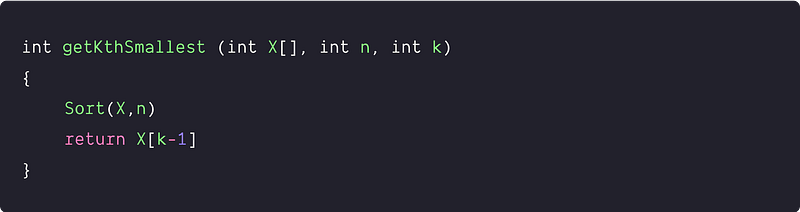Time and Space complexity analysis

Suppose we are using efficient O(nlogn) sorting algorithm heap sort. Time Complexity = Time Complexity of the heap sort + Time Complexity of accessing kth smallest element = O(nlogn) + O(1) = O(nlogn)

Space Complexity: O(1) because heap sort is an in-place sorting algorithm.

### Using the min-heap data structure

Solution Idea and Steps

Min heap is an array-based complete binary tree structure where the value in each node is smaller than or equal to the values in the children of that node. So minimum element is always present at the root, i.e., X. We use the min-heap for the efficient implementation of a min-priority queue. Here are some critical min-heap operations:

• getMin(): Provide fast access to the minimum element in O(1).
• deleteMin(): Remove minimum element in O(logn).
• Insert(): Add an element in O(logn).
• We can build a min-heap in O(n) using the bottom-up approach.

So how do we optimize time complexity using min heap? Here is an idea:

• Build a min-heap of all n array elements.
• Remove k - 1 smallest element by continuously performing the deleteMin() operation on the min-heap.
• After this, the kth smallest element will be present on the root. We can access this in O(1) time complexity by calling the getMin() operation.

Here we have provided the complete class-based implementation of the min-heap.

Solution Pseudocode

Finding the kth smallest element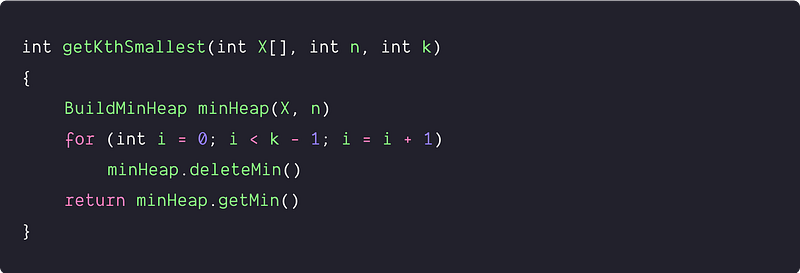Min heap implementation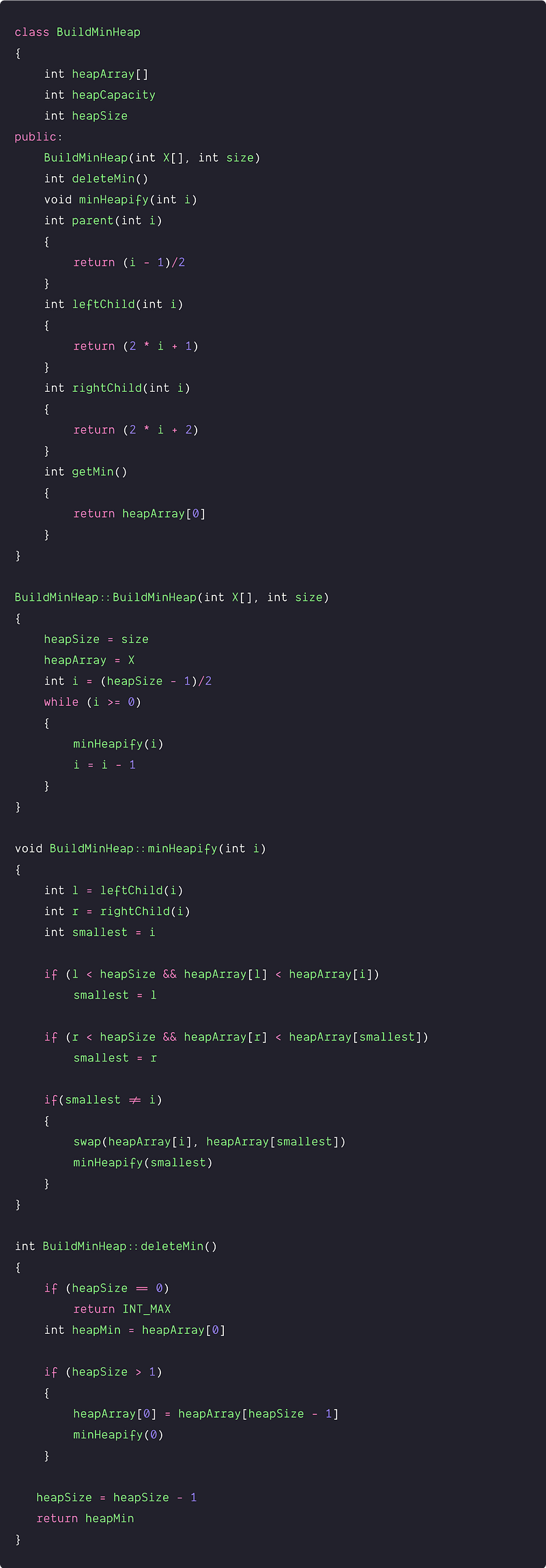Time and space complexity analysis

Time Complexity = Time complexity of building the min-heap size n + Time complexity of deleting min element k-1 times + Time complexity of accessing the kth min element.

• The time complexity of building the min-heap size n = O(n)
• After each deletion the size of the min heap will reduce by 1. So time complexity of deleting min element k-1 times = log n + log (n - 1) + log (n - 2)....+ log (n - k - 1) = log (n * (n-1)....*(n-k-1)) < log(n^ (k-1)) = (k-1) logn = O(klogn). Here time complexity depends on the value of k (1 <= k <= n).
• The time complexity of accessing the kth min element = O(1)

So overall time complexity = O(n) + O(klogn) + O(1) = O( n + klogn)

• Best case occur if k = 1, time complexity = O(n + logn) = O(n)
• Worst case occur if k = n, time complexity = O(n + nlogn) = O(nlogn)

Space Complexity = O(1) because we build min-heap in place using the same array. So we are using constant memory. Now a critical question would be: can we improve optimize the solution further?

### Using the max-heap data structure

Solution Idea

Similar to min-heap, the max heap is an array-based complete binary tree structure where the value in each node is larger than or equal to the values in the children of that node. So maximum element is always present at the root, i.e., X. We use max-heap for the efficient implementation of a max-priority queue. Here are some critical max-heap operations:

• getMax(): Provide fast access to the maximum element in O(1).
• deleteMax(): Remove maximum element in O(logn).
• Insert(): Add an element in O(logn).
• We can build a max-heap in O(n) time complexity using the bottom-up approach.

How do we solve this problem using max heap? A solution insight would be: If we have a max-heap of the k smallest elements, then the kth smallest element will be present at the root of the heap and we can return the root value in O(1). But the critical question would be - how do we generate the max heap of k smallest element? Let's think.

Solution Steps

• We start with building a max-heap of the first k elements of the array. There is no need to allocate extra space, and we can use the same array from index i = 0 to k-1. After this process, the max element of the first k element will be present at the root of the heap, i.e., X.
• Now the idea is to track the k smallest elements using the k size max heap. For this, we dynamically update the max heap with the smaller values (values smaller than the root of the max heap) present in the array from the index k to n-1.
• In other words, we run a loop from i = k to n -1, check If (X[i] < root), then we replace the max heap root with X[i] and run heapify() operation on the root to maintain the max heap property. Otherwise, we ignore the element and move on. Here we access the root value by the getMax() function and replace the heap root by replaceMax(X[i]) function.
• By the end of the above loop, the max heap contains the top k smallest element of the array i.e. kth smallest element will be present at the root. We return this value.

Solution Pseudocode

Finding the kth smallest element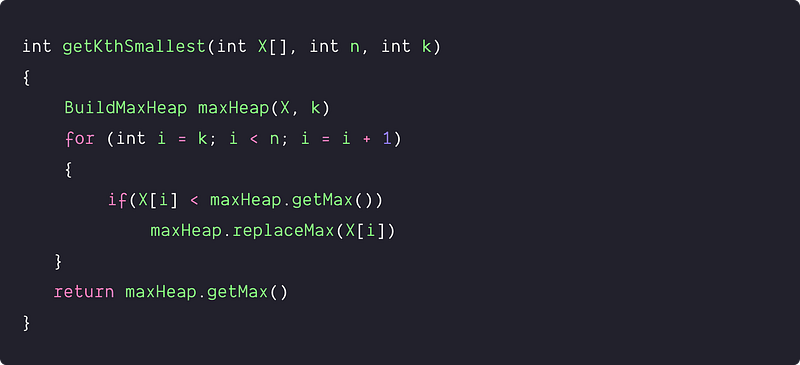Max heap implementation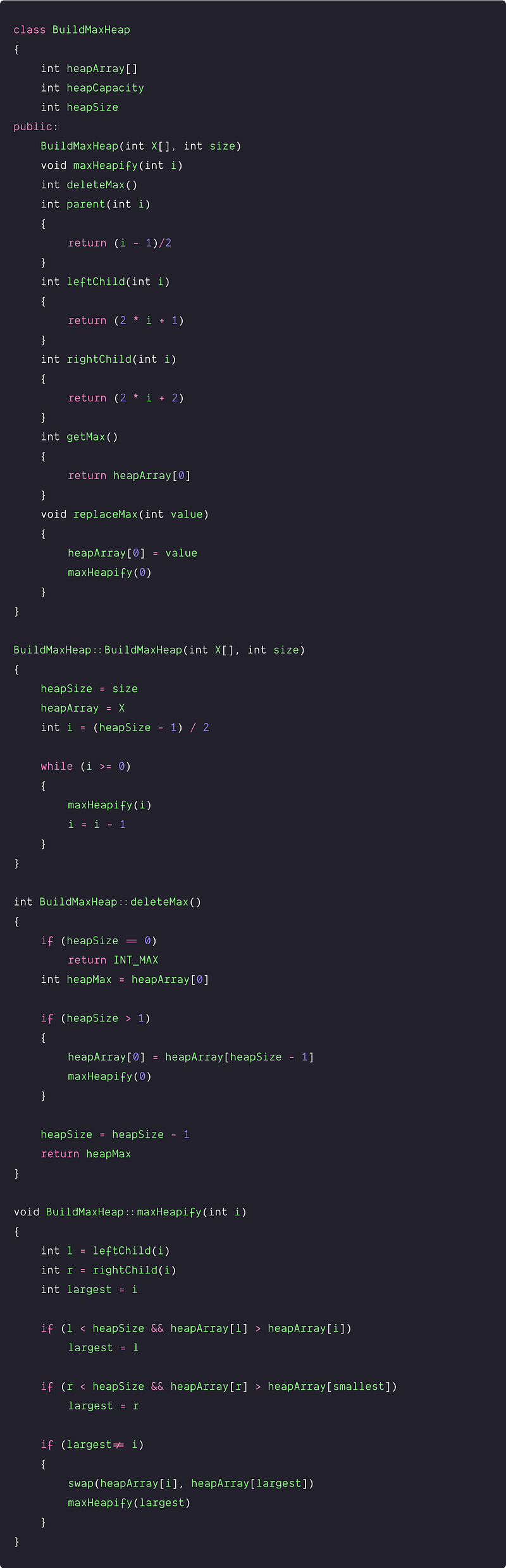Time and space complexity analysis

The time complexity of building k size max-heap = O(k).

Now in the worst case, at each iteration of the loop, if(X[i] < maxHeap.getMax()) will be true and we execute maxHeap.replaceMax(X[i]) operation every time. The time complexity of each replaceMax() on k size heap = O(log k). So the overall time complexity of the (n -k) replaceMax() operation = (n-k)*O(log k) = O((n-k)log k)

Time Complexity in the worst case = O(k) + O((n-k)*logk) + O(1) = O(k + (n-k)*logk) = O(k + nlogk - klogk) = O(nlogk), k and klogk are lower order terms.

Space Complexity = O(1), we are not using any extra space.

### Quick-select approach: Divide and conquer idea similar to quick-sort

Solution Idea

Now we are going to discuss an interesting quick select approach that solves the problem efficiently by using a divide and conquer idea similar to the quick sort.

The solution intuition comes from the quick-sort partition process, which divides the array into two parts around a pivot and returns the index of the pivot in the sorted array. Elements in the array will look like this after the partition:

X [ l to pos - 1 ] < pivot < X [ pos + 1.....r ]

The pivot element is present at index pos, and pos - l elements are smaller than the pivot. So pivot element is (pos - l + 1)th smallest element in the array.

• if ((pos - l + 1) == k) then pivot is the kth smallest and we return X[pos].
• if ((pos - l + 1) > k) then the kth smallest must be present in the left subarray.
• if ((pos - l + 1) < k) then the kth smallest must be present on the right subarray.

So from the above insight, we can develop an approach to use the partition process to find the kth smallest element recursively. But unlike the quick-sort, which processes both subarrays recursively, we process only one subarray recursively. We recur for either the left or right side based on the comparison with k and the position of the pivot.

Solution Steps

• We take the function kthSmallest(int X[], int l, int r, int k), where the left and right ends of the array are input. Initially l = 0 and r = n-1.  The base case is the scenario of single-element array i.e if (l ==r), return X[l].
• Now we partition the array X[l … r] into two subarrays (X[l … pos-1] and X[pos + 1 ... r] ) using the partition process similar to the quick sort. Here pos is the index of the pivot returned by the partition process.
• After the partition, we calculate the pivot order in the array, i.e., i = pos - l + 1. Pivot is the (pos - l + 1)th smallest element.
• if (i == k), then we return X[pos]. Otherwise determines in which of the two subarrays X[l .. pos-1] and X[pos + 1 .. r] the kth smallest is present.
• If (i > k), then the desired element must lie on the left subarray. We call the same function recursively for the left part, i.e., kthSmallest(X, l, pos-1, k).
• If (i < k), then the desired element must lie on the right subarray. We call the same function recursively for the right part i.e, kthSmallest(X, pos+1, r, k-i). Note — The desired element is the (k-i)th smallest element of the right side because we already know the i number of values smaller than the kth smallest element i.e. left subarray and a pivot.

Solution Pseudocode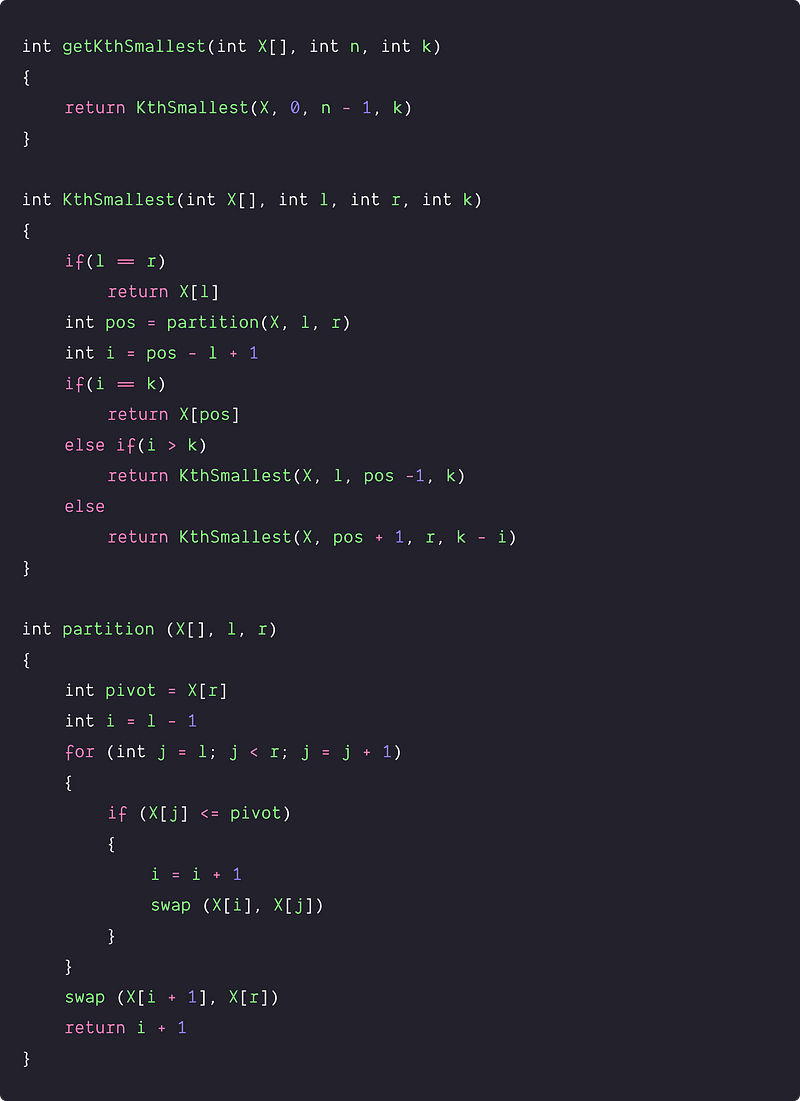Time and space complexity analysis of the quick-select

This is a divide and conquers algorithm, where we are solving only one subproblem.

Worst-case analysis: Worst case situation occurs when the partition is bad and highly unbalanced. There would be two scenarios: either 0 elements in the left subarray and (n - 1) elements in the right subarray or (n - 1) elements in the left subarray and 0 elements in the right subarray. So in the worst case, the algorithm always explores the larger sub-problem of size n - 1.

• For the partition algorithm, we are running a single loop with constant operation at each iteration. So the time complexity of the partition algorithm = O(n)
• So worst case time complexity T(n) = Time complexity of the partition algorithm + Worst case time complexity of the one subproblem + Time complexity of extra operations = O(n) + T(n-1) + O(1) = T(n - 1) + O(n) = T(n - 1) + cn
• The solution of the above recurrence is O(n^2). We can easily solve the above recurrence using the recursion tree method. Refer to the worst-case analysis of quicksort.

The quick select algorithm looks highly inefficient in the worst case. But the real magic would be average case analysis because the algorithm works in O(n) time complexity average. How? Let's think.

Average case analysis: We assume that the partition process chooses pivot randomly. In other words, all possibility of partition is equally likely and the probability of occurring is the worst case in 1/n.

• Input size of the left subproblem = i - 1, Input size of the left subproblem = n - i. Here the value of i can be in the range of 1 to n. The time complexity of the left subproblem = T(i - 1), Time complexity of the left subproblem = T(n - i).
• We solve only one subproblem recursively, so let's take the upper bound: the time needed for the recursive call on the largest possible input. In other words, to obtain an upper bound, we assume that the kth smallest element is always on the side of the partition with the greater number of elements. So time complexity of the smaller subproblem = T(max (i - 1, n - i)), where 1<= i <= n.
• For calculating th average case, we explore all n possible values of T(max (i - 1, n - i)) and divide it by n. Recurrence relation for the average case:

T(n) = 1/n * [ i = 1 to n ∑ T(max (i - 1, n - i)) ] + O(n)

• if i > n/2, max (i - 1, n - i) = i - 1
• if i <= n/2, max (i - 1, n - i) = n - i

So from i = 1 to n, each term T(i) appears twice in the above formula (Think!). We cab also place cn in place of O(n). So we can simplify the above formula.

T(n) <= 2/n [ i = n/2 to n-1 ∑ T(i)) ] + cn

We can solve this using the “guess and check” or substitution method based on our intuition. Let's assume that T(n) is a linear function, i.e., T(n) ≤ pn, where p is a constant.

T(n) <= 2/n ( i = n/2 to n-1 ∑ pi ) + cn

Let's further simplify the expression on the right-hand side

``````2/n (i = n/2 to n-1 ∑pi) + cn
= 2p/n (i = n/2 to n-1 ∑ i) + cn
= 2p/n [( i = 0 to n-1 ∑ i) - (i = 0 to n/2-1 ∑ i)] + cn
= 2p/n [n(n-1)/2 - (n/2 - 1)(n/2 - 2)/2] + cn
= p/n [n(n-1) - (n/2 - 1)(n/2 - 2)] + cn
= p/n [n^2 - n - n^2/4 + n + n/2 - 2] + cn
= p/n [3n^2/4 + n/2 - 2] + cn
= p [3n/4 + 1/2 - 2/n] + cn
= 3pn/4 + p/2 - 2p/n + cn
= pn - (pn/4 - cn - p/2)
``````

In order to complete the proof, we need to show that for sufficiently large n, pn - (pn/4 - cn - p/2) is at most pn or, equivalently (pn/4 - cn - p/2) > 0 => n(c- p/4) > p/2 => n > (p/2)/(c- p/4)

(p/2)/(c- p/4) is a small constant, so our guess would be correct for n > (p/2)/(c- p/4). So average case time complexity of quick select algorithm T(n) = pn = O(n)

Space complexity: Worst case situation occur when the partition is bad and the height of the recursion tree will be O(n). In such a scenario, recursion decreases by 1 and allocates O(n) stack space. But in the average case, space complexity would be O(logn). (Think!)

### Critical ideas to think!

• Can we improve the time complexity of the 4th approach to O(n) in the worst-case scenario? Explore the median finding algorithm for finding the kth smallest element.
• How do we modify the above algorithms to handle duplicates?
• In the 1st approach, do we need to sort the entire array?
• What would be other ways to perform the partition of an array?
• Why average case space complexity is O(logn) in the 4th approach?
• Can this problem be solved using a Binary Search Tree? What would be the complexity in that case?
• Why is Heap preferred over BST for the Priority Queue implementation?
• In the 3rd approach, why do we ignore elements larger than root in the second part of the algorithm? What would be the worst and best case input?

### Comparisons of time and space complexities

• Using sorting: Time = O(nlogn), Space = O(1)
• Using min-heap: Time = O(n + klogn), Space = O(1)
• Using max-heap: Time = O(nlogk), Space = O(1)
• Using Quick-Select: Time = O(n) average, Space = O(logn) average

### Similar coding questions to practice

• Find the Kth largest element.
• Find k smallest elements in an array.
• Median of two sorted arrays of the same size
• The kth missing element in an unsorted array
• Find the kth largest element in a stream.
• kth Smallest Element in a Sorted Matrix
• k smallest elements in an array
• kth element of two sorted Arrays
• Find the top k frequent elements.

Enjoy learning, Enjoy coding, Enjoy algorithms!

Get well-designed application and interview centirc content on ds-algorithms, machine learning, system design and oops. Content will be delivered weekly.